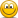# Java Computer Science

Discussion in 'School Work Help' started by Woody, Sep 2, 2010.

1. ### Woody Well-Known Member

213
41
0
I copied the code from the pdf to my editor and tried running it and I got a few errors

Code:
```C:\Computer Science 1020\Test Examples>javac Eg.java
Eg.java:3: non-static variable this cannot be referenced from a static context
{ Student s1 = new Student("John",202123456);
^
Eg.java:5: non-static variable this cannot be referenced from a static context
^
Eg.java:44: cannot find symbol
symbol  : constructor Student()
location: class Eg.Student
{
^
3 errors```
Code:
```public class Eg
{ public static void main(String[] args)
{ Student s1 = new Student("John",202123456);
System.out.println(s1.toString());//calls Student’s
}

public class Student
{
private String name;
private long number;

public Student(String aName, long aNumber)
{ this.setName(aName);
this.setNumber(aNumber);
}

public void setName(String aName)
{ this.name = aName;
}
public String getName()
{ return this.name;
}
public void setNumber(long aNumber)
{ this.number = aNumber;
}
public long getNumber()
{ return this.number;
}
public String toString()
{ return "Student[name=" + this.getName() +
",number=" + this.getNumber() + "]";
}
}

{
private String major;
private int year;

public Undergrad(String aName, long aNumber,String aMajor, int aYear)
{
}

public void setMajor(String aMajor)
{ this.major = aMajor;
}
public String getMajor()
{ return this.major;
}
public void setYear(int aYear)
{ this.year = aYear;
}
public int getYear()
{ return this.year;
}
public String toString()
{ return "Undergrad[name=" + this.getName() +",number=" + this.getNumber() +",major=" + this.getMajor() +",year=" + this.getYear() + "]";
}
}
}```

I'm not really good at computer science but I'm still trying my best.
Can someone correct the mistakes?
Thank you.

#1
Last edited: Sep 2, 2010
2. pdf? did you dl or is it yours? lol

#2
3. ### -Tisken- Well-Known Member

uhm.... im not sure if you can put all three classes in one file. i used to separate them.

Code:
```public class Eg
{
public static void main(String[] args)
{
Student s1 = new Student("John",202123456);
System.out.println(s1.toString());//calls Student’s
}
}
```
Code:
```public class Student
{
private String name;
private long number;

//Constructor
public Student(String aName, long aNumber)
{
this.setName(aName);
this.setNumber(aNumber);
}

//Set-Methode
public void setName(String aName)
{
this.name = aName;
}

public void setNumber(long aNumber)
{
this.number = aNumber;
}
//Get-Methode
public String getName()
{
return this.name;
}

public long getNumber()
{
return this.number;
}
public String toString()
{
return "Student[name=" + this.getName() +
",number=" + this.getNumber() + "]";
}
}
```
Code:
```
{
private String major;
private int year;

public Undergrad(String aName, long aNumber,String aMajor, int aYear)
{
[COLOR="#ff0000"]		super(aName, aNumber);
this.major = aMajor;
this.year = aYear;[/COLOR]
}

public void setMajor(String aMajor)
{
this.major = aMajor;
}
public String getMajor()
{
return this.major;
}
public void setYear(int aYear)
{
this.year = aYear;
}
public int getYear()
{
return this.year;
}
public String toString()
{
return "Undergrad[name=" + this.getName() +",number=" + this.getNumber() +",major=" + this.getMajor() +",year=" + this.getYear() + "]";
}
}
```4. ### Woody Well-Known Member

213
41
0
That doesn't really matter. :nono:

#4
5. ### Woody Well-Known Member

213
41
0

Danke -Tisken-.

Now I have to understand this. lol -worship

#5
6. ### -Tisken- Well-Known Member

what you dont understand?7. ### Woody Well-Known Member

213
41
0
I think I get it now.
I'm just looking at some notes that I'll be studying this school term.-cool

#7